Courses

# Ex 6.4 NCERT Solutions- Triangles Class 10 Notes | EduRev

## Class 10 : Ex 6.4 NCERT Solutions- Triangles Class 10 Notes | EduRev

The document Ex 6.4 NCERT Solutions- Triangles Class 10 Notes | EduRev is a part of the Class 10 Course Class 10 Mathematics by VP Classes.
All you need of Class 10 at this link: Class 10

Q.1. Let ΔABC ~ ΔDEF and their areas be, respectively, 64 cm2 and 121 cm2. If EF = 15.4 cm, find BC.
Sol. Since, ΔABC ~ ΔDEF The ratio of the areas of two similar triangles is equal to the ratio of the squares of the corresponding sides,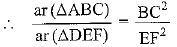⇒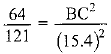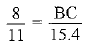⇒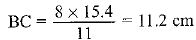Q2. Diagonals of a trapezium ABCD with AB || DC intersect each other at the point O. If AB = 2 CD, find the ratio of the areas of triangles AOB and COD.
Sol. ABCD is a trapezium with
AB || DC and AB = 2 CD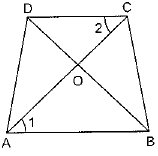In ΔAOB and ΔCOD,
∠AOB = ∠COD   [V.O.A.]
∠1 = ∠2 [Alternate angles]
∴ ΔAOB ~ ΔCOD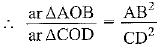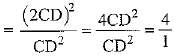⇒ ar ΔAOB: ar ΔCOD = 4 : 1

Q.3. In the figure, ABC and DBC are two triangles on the same base BC. If AD intersects BC at O, show that.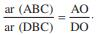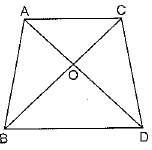Sol. ABC and DBC are two triangles on the same base BC.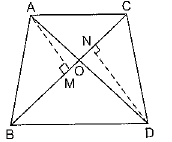Construction: Draw AM ⊥ BC and DN ⊥ BC
Proof: In ΔAOM and ΔDON
∠AOM = ∠DON   [V.O.A.]
∠AMO = ∠DNO    [Each 90°]
∴  ΔAOM ~ ΔDON    [AA]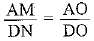[Corresponding sides of similar triangles]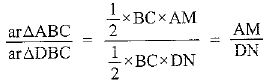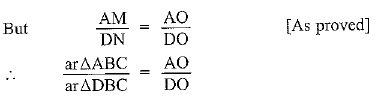Q.4. If the areas of two similar triangles are equal, prove that they are congruent.
Sol.
Given: Consider ΔABC ~ ΔDEF
and ar ΔABC = ar ΔDEF
To Prove; ΔAABC ≌ ΔDEF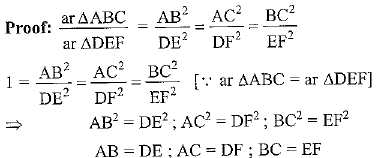∴ ΔABC ≌ ΔDEF [By SSS congruency rule]

Q.5. D, E and F are respectively the mid-points of sides AB, BC and CA of D ABC. Find the ratio of the areas of ΔDEF and ΔABC.
Sol. Given: D, E and F are mid-points of sides BC, CA and AB respectively.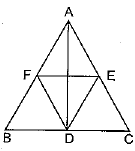Proof: D and E are mid-points of sides BC and CA respectively
∴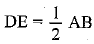[Line segment joining the mid-points of two sides of triangle is parallel to the third side and half of it.]
Similarly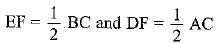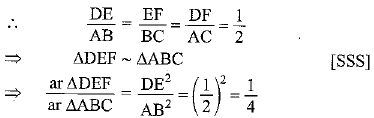Hence ar ΔDEF : ar ΔABC = 1 : 4

Q.6. Prove that the ratio of the areas of two similar triangles is equal to the square of the ratio of their corresponding medians.
Sol.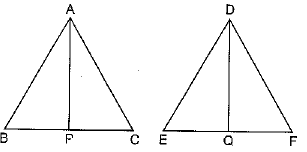Given: ΔABC ~ ΔDEF, AP and DQ are medians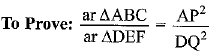Proof: The ratio of the areas of two similar triangles is equal to the ratio of squares of two corresponding side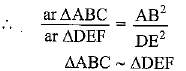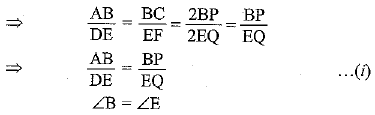[Corresponding angles of similar triangle]
∴ ΔABP ~ ΔDEQ       [SAS]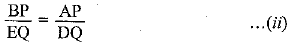From equation (i) and (ii)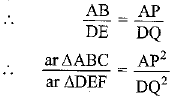Q.7. Prove that the area of an equilateral triangle described on one side of a square is equal to half the area of the equilateral triangle described on one of its diagonals.
Sol. Let the side of the square ABCD be a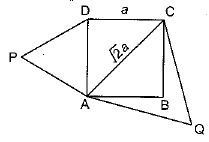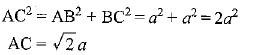ΔPAD ~ ΔQAC    [AA similarity each angle = 60°]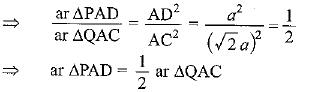Q.8. ABC and BDE are two equilateral triangles such that D is the mid-point of BC. Ratio of the areas of triangles ABC and BDE is
(A) 2:1
(B) 1:2
(C) 4:1
(D) 1:4
Sol. Justification: Let AB = BC = CA = a
D is the mid point of BC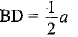ΔABC ~ ΔBDE
[Given that they are equilateral triangles]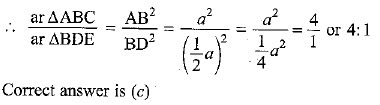Q.9. Sides of two similar triangles are in the ratio 4:9. Areas of these triangles are in the ratio
(A) 2:3
(B) 4:9
(C) 81:16
(D) 16:81
Sol. Justification: Areas of two similar triangles are in the ratio of the squares of their corresponding sides.
∴  Ratio of areas of triangle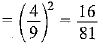or  16:81

Offer running on EduRev: Apply code STAYHOME200 to get INR 200 off on our premium plan EduRev Infinity!

132 docs

,

,

,

,

,

,

,

,

,

,

,

,

,

,

,

,

,

,

,

,

,

;